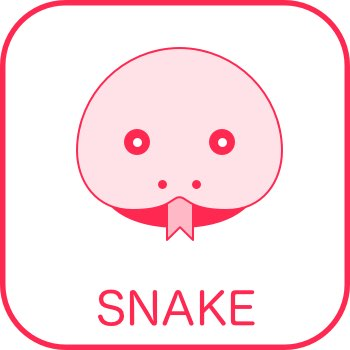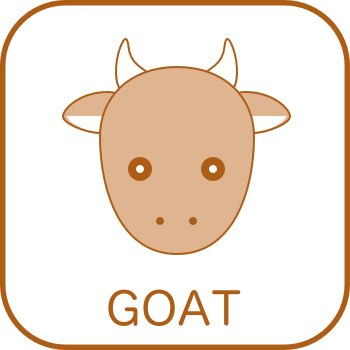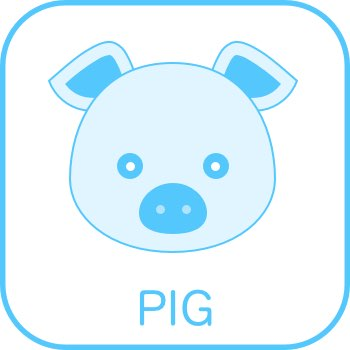skip to Main Content

# FIVE ELEMENT & TALENT ANALYSISNAME: Ivona
GENDER: Female
MASTER ELEMENT (ME): Yang Water
DATE OF BIRTH: 2007-07-07
LOCAL TIME OF BIRTH: 10:
SOLAR TIME OF BIRTH:

Birth Chart (also known as BaZi Chart) represents the energy matrix which was active at that exact point of time. It contains Elements on top and Branches at the bottom. While Elements are a pure manifestation of one particular Element, Branches are mostly packages of various Elements. There are altogether over 10 million possible chart combinations.

### HOUR### DAY (ME)### MONTH### YEARLearn how this analysis works

Warning: Hour of birth is missing, the analysis might lack certain data
Warning: Date of birth is close to month change, make sure that time of birth is exact to avoid possible mistakes
Warning: Time of birth is close to hour change, make sure that time of birth is exact to avoid possible mistakes

#### MASTER ELEMENT (ME)

Master Element or ME is the central point of reference. Each ME has its own character traits, but those might vary a lot, depending on overall chart conditions. Generally speaking, if ME is weak or feeble, it needs those Elements which can make it stronger. If ME is strong or dominant, it needs elements which will challenge it. If ME is solid, in most cases it represents the ability to handle all Elements without major disturbances.

FEEBLE WEAK STRONG EXCESSIVE SOLID

#### FIVE ELEMENTS

Five Elements values are generated from the Birth Chart and represent the general dynamic between them.

<!–

#### "CHARACTER

Character Components are generated from Five Elements, with a certain level of modifications. They represent a basic potential of the Chart, but whether this potential can be reached has to be analyzed in detail in Talent Analysis.

Body Creativity Wealth Power Mind –>

#### TOP TALENTS

These are the strongest Talents in this chart. Like with Character Components, they are just potentials, but whether the person will be able to access and obtain them, will depend on overall chart condition. This will be analyzed further below.

Coming soon…

Coming soon…

#### TALENTS ANALYSIS

Coming soon…

BETA DISCLAIMER – WORK IN PROGRESS

// ROTATE MASTER ELEMENT GRAPH var rot = 33.025-90; TweenMax.set(‘#polymeter’, { transformOrigin:”100px 690px”} ); TweenMax.to(‘#polymeter’, 1, {rotation: rot }) ; // CHARACTER STAR var JJ = {“Body”:8.28,”Creativity”:32.49,”Wealth”:36.51,”Power”:19.94,”Mind”:2.76}; var cc = []; var template={“Meta”:0,”Creativity”:0,”Wealth”:0,”Power”:0,”Mind”:0}; for(one in JJ){ template[one] = JJ[one] ; } for(one in template){ cc.push( template[one] ); } // math helper… function V2P(value, index, total) { var x = 0; var y = -value * 0.8; var angle = Math.PI * 2 / total * index; var cos = Math.cos(angle); var sin = Math.sin(angle); var tx = x * cos – y * sin + 100; var ty = x * sin + y * cos + 100; var ret = { x: tx, y: ty, lx: 0 * cos + 90 * sin + 100, // labels x & y coords ly: 0 * sin – 90 * cos + 100 }; return ret; } // genere star for user! var o = cc.map( (x, i) => V2P(x,i,5).x+’,’+V2P(x,i,5).y ); jQuery(‘#CC’).attr( ‘points’, o.join(‘ ‘) ); // 5 ELEMS var myCanvas = document.getElementById(“myFiveElemCanvas”); myCanvas.width = 1000; myCanvas.height = 1000; var ctx = myCanvas.getContext(“2d”); function drawPieSlice(ctx,centerX, centerY, radius, startAngle, endAngle, color ){ ctx.fillStyle = color; ctx.beginPath(); ctx.moveTo(centerX,centerY); ctx.arc(centerX, centerY, radius, startAngle, endAngle); ctx.closePath(); ctx.fill(); } // class to render pie charts var Piechart = function(options){ this.options = options; this.canvas = options.canvas; this.ctx = this.canvas.getContext(“2d”); this.colors = options.colors; this.draw = function(){ var total_value = 0; var color_index = 0; for (var categ in this.options.data){ var val = this.options.data[categ]; total_value += val; } var start_angle = 0; for (categ in this.options.data){ val = this.options.data[categ]; var slice_angle = 2 * Math.PI * val / total_value; drawPieSlice( this.ctx, this.canvas.width/2, this.canvas.height/2, Math.min(this.canvas.width/2,this.canvas.height/2), start_angle, start_angle+slice_angle, this.colors[color_index%this.colors.length] ); start_angle += slice_angle; color_index++; } //drawing a white circle over the chart //to create the doughnut chart if (this.options.doughnutHoleSize){ drawPieSlice( this.ctx, this.canvas.width/2, this.canvas.height/2, this.options.doughnutHoleSize * Math.min(this.canvas.width/2,this.canvas.height/2), 0, 2 * Math.PI, “#ffffff” ); } start_angle = 0; for (categ in this.options.data){ val = this.options.data[categ]; slice_angle = 2 * Math.PI * val / total_value; var pieRadius = Math.min(this.canvas.width/2,this.canvas.height/2); var labelX = this.canvas.width/2 + (pieRadius / 2) * Math.cos(start_angle + slice_angle/2); var labelY = this.canvas.height/2 + (pieRadius / 2) * Math.sin(start_angle + slice_angle/2); if (this.options.doughnutHoleSize){ var offset = (pieRadius * this.options.doughnutHoleSize ) / 2; labelX = this.canvas.width/2 + (offset + pieRadius / 2) * Math.cos(start_angle + slice_angle/2); labelY = this.canvas.height/2 + (offset + pieRadius / 2) * Math.sin(start_angle + slice_angle/2); } var labelText = Math.round(100 * val / total_value); this.ctx.fillStyle = “white”; this.ctx.font = “50px Arial”; this.ctx.fillText(categ.toUpperCase(), labelX-50,labelY); this.ctx.fillText(labelText+”%”, labelX-50,labelY + 60); start_angle += slice_angle; } if (this.options.legend){ color_index = 0; var legendHTML = “”; for (categ in this.options.data){ legendHTML += “
“+categ+”
“; } this.options.legend.innerHTML = legendHTML; } } } var my5Els ={“fire”:11.9,”earth”:6.5,”metal”:0.9,”wood”:10.59,”water”:3.79}; var myDougnutChart = new Piechart( { canvas:myCanvas, data:my5Els, colors:[‘#ad5d16′,’#ff9600′,’#54c7fc’,’#44db5e’,’#fe2851′], doughnutHoleSize:0.5, // legend:myLegend } ); myDougnutChart.draw();

Close search